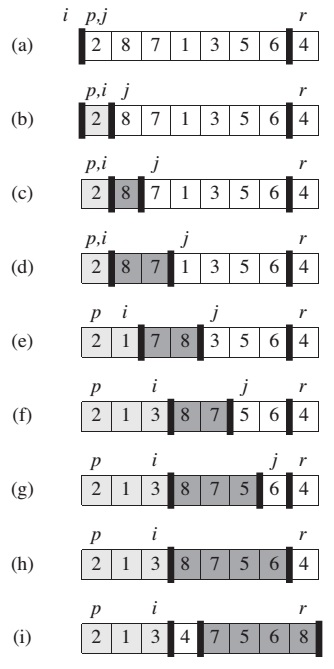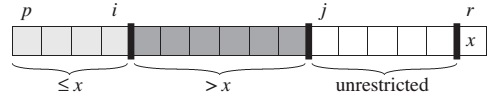The quicksort algorithm has a worst-case running time of O(n^2) on an input array of n numbers, and worst case happens when the array is sorted in descending order. Despite a worst-case running time, quicksort is often the best practical choice for sorting. Its average expected running time is O(n log n), and it has an advantage of sorting in place.

## Description of Quicksort

• Divide: Parititon the array A[p..r] into two sub-arrays A[p..q-1] and A[q+1..r] such that each element of A[p..q-1] <= A[q] <= A[q+1..r]
• Conquer: Sort the two subarrays A[p..q-1] and A[q+1..r] by recursive calls to quicksort
• Combine: Because the subarrays are already sorted, no work is needed to combine them
``````QUICKSORT(A, p, r):
if p < r
q = PARTITION(A, p, r)
QUICKSORT(A, p, q - 1)
QUICKSORT(A, q + 1, r)
``````

Initial call: QUICKSORT(A, 1, A.length)

### Partitioning the array

``````PARTITION(A, p, r)
x = A[r]
i = p - 1
for j = p to r - 1
if A[j] <= x
i = i + 1
exchange A[i] with A[j]
exchange A[i + 1] with A[r]
return i + 1
``````As a procedure runs, it partitions the array into four regions.Best-case partitioning occurs when PARTITION produces two subproblems, each of size no more than n/2, since one is of size floor(n/2) and one of size ceil(n/2)-1. In this case quicksort runs much faster, with recurrence time:

T(n) = 2T(n/2) + O(n)

this recurrence has the solution T(n) = O(n lg n) Average-case running time of quicksort is much closer to the best case than to the worst case.

## A Randomized version of quicksort

Instead of always using A[r] as the pivot, we will select a randomly chosen element from the subarray A[p..r]. We do so by first exchanging element A[r] with an element chosen at random from A[p..r]. By randomly sampling the range p,…,r we ensure that the pivot element x = A[r] is equally likely to be any of the r-p+1 elements in the subarray.

``````RANDOMIZED-PARTITION(A, p, r)
i = RANDOM(p, r)
exchange A[r] with A[i]
return PARTITION(A, p, r)
``````

Randomized look of the quicksort will be as follows:

``````if p < r
q = RANDOMIZED-PARTITION(A, p, r)
RANDOMIZED-QUICKSORT(A, p, q-1)
RANDOMIZED-QUICKSORT(A, q+1, r)
``````

The quicksort procedure was invented by Hoare. Hoare’s version appears in the “Introduction to Algorithms” book [Cormen] at Problem 7-1.